# Two vans

At 8:30 a. M. , two vans leave at a parking area. They travel in opposite directions: one van travels at 48 km/h and the other at 62 km/h. At what time will the cars be 550 km apart?

Result

t = 13:30 hh:mm#### Solution:

$s = 550 \ km \ \\ v_{ 1 } = 48 \ km/h \ \\ v_{ 2 } = 62 \ km/h \ \\ \ \\ v = v_{ 1 }+v_{ 2 } = 48+62 = 110 \ km/h \ \\ \ \\ t_{ 1 } = s/v = 550/110 = 5 \ h \ \\ \ \\ t = t_{ 1 } + (8+30/60) = 5 + (8+30/60) = \dfrac{ 27 }{ 2 } = 13.5 = 13:30 \ \text{ hh:mm }$Our examples were largely sent or created by pupils and students themselves. Therefore, we would be pleased if you could send us any errors you found, spelling mistakes, or rephasing the example. Thank you!

Leave us a comment of this math problem and its solution (i.e. if it is still somewhat unclear...):Be the first to comment!Tips to related online calculators
Do you want to convert length units?
Do you want to convert velocity (speed) units?
Do you want to convert time units like minutes to seconds?

## Next similar math problems:

1. Inter city busThe inter city bus leaves Suva at 10.00am and reaches Nadi at 1.00pm covering a distance of 219km. How long did it take to reach the bus Nadi?
2. Cyclist 9A cyclist travels at a a speed of 4.25 km per hour. At that rate, how far can he travel in 3.75 hours?The railroad runs parallel to the railway. The train travels at 36 km/h on track. In the opposite direction, the motorcycle rides at 90 km/h. The train passes in 3 seconds. How long is a train?
4. Chase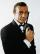Paul travel at 60 km/h. After 18 km ride found that he forgot an important book. His brother Thomas behind him carrying it. How fast must Thomas go to catch up with Paul in 31 minutes?
5. Bus 14Boatesville is 65.35 kilometers from Stanton. A bus traveling from Stanton is 24.13 kilometers from Boatesville. How far has the bus traveled?
6. Train and walker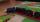Walker passes an hour 5 km. Express train per hour 100 km. Walker went 4 hours. How many minutes took the distance express train?
7. Knight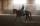Knight passed 13 km long track in 26 minutes, what was his average speed?
8. Pedestrian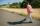Dana started at 10:00 from the point A to point B. These points are distant 12 km. Determine how fast Daniel went when the place B arrived at 11:54. Speed express in km/h.
9. Average speedMichal went out of the house by car at speed 98 km/h. He came into the goal place for 270 minutes. Determine the distance between the two places.
10. Constant motionThe car has undergone constant motion 120 km in 1 hour 30 minutes. Determine its average speed. Determine how long car drove in the first 20 minutes and 50 minutes from the start of the move.
11. The cyclistThe cyclist drove from A to B, 60 km away. The first half drove at 30km/h, the second at 20km/h. How long did it take for him?
12. Cyclist and carA cyclist who runs at a speed of 20km per hour will arrive at the finish in 4.5 hours. How fast does the car have to drive the same route in 2 hours?
13. Speed of sound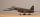The average speed of sound is 330 meters per second. Estimate how long it will hear the church bell 1 km away. Calculate the distance from what would hear sound after 10 seconds.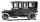Car started at 11:40 at speed 54 km/h from A and to B arrived at 12:20. Determine the distance A and B.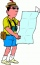Lucie can walk about 3 4/5 miles each hour. How far can she walk in 2 hours 45 minutes?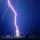There was a glimpse of the sky and we hear a thunder in 4.5 seconds. The light spreads at 300,000 kilometers per second, so we can assume the flash instantly without delay. However, the speed of the sound is much smaller in the air one third of a kilomete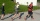The toy car runs at an average speed of 2 m/s. In a minute, it will pass the entire circuit five times. How long is the loop?